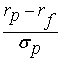### Why should I choose AnalystNotes?

Simply put: AnalystNotes offers the best value and the best product available to help you pass your exams.

##### Subject 8. The Sharpe Measure of Risk-Adjusted Performance
The Sharpe Measure is a more precise return-risk measure than standard deviation. It recognizes the existence of a risk-free return, a return for virtually zero standard deviation. It measures the reward to total volatility trade-off.

It is defined as:• (rp - rf) = the extra reward that investors receive for the added risk taken, called the excess return on portfolio p
• σp = the standard deviation of the portfolio returns

Note that the numerator of the Sharpe measure recognizes the existence of a risk-free return. Portfolios with large Sharpe ratios are preferred to those with smaller ratios because we assume that investors prefer return and dislike risk. The Sharpe ratio is also called the reward-to-variability ratio.

Example

The mean monthly return on T-bills (the risk-free rate) is 0.25%. The mean monthly return on the S&P 500 is 1.30% with a standard deviation of 7.30%. Calculate the Sharpe measure for the S&P 500 and interpret the results.

Sharpe measure = (1.30 - 0.25)/7.30 = 0.144
Interpretation: The S&P 500 earned 0.144% of excess return per unit of risk, where risk is measured by standard deviation.

Learning Outcome Statements

i. calculate and interpret the coefficient of variation and the Sharpe ratio;

CFA® Level I Curriculum, 2020, Volume 1, Reading 7

User Comment
jehangir Sharpes measure is more suerior to sd and measures excess return per unit of risk which is measured by standard deviation
loisliu88 except when Sharpe ratio is negative, it doesn't work
mohsindi How come it recognize Risk free return ? the formula deducts the Rf from rp
choas69 it recognize the existence of risk free thus excluding it out of the formula to compute only the returns associated with risk, the bigger the results the more the better the assist.
Why the S&P 500 earned 0.144% of excess return in the example? Why not 14.4%?